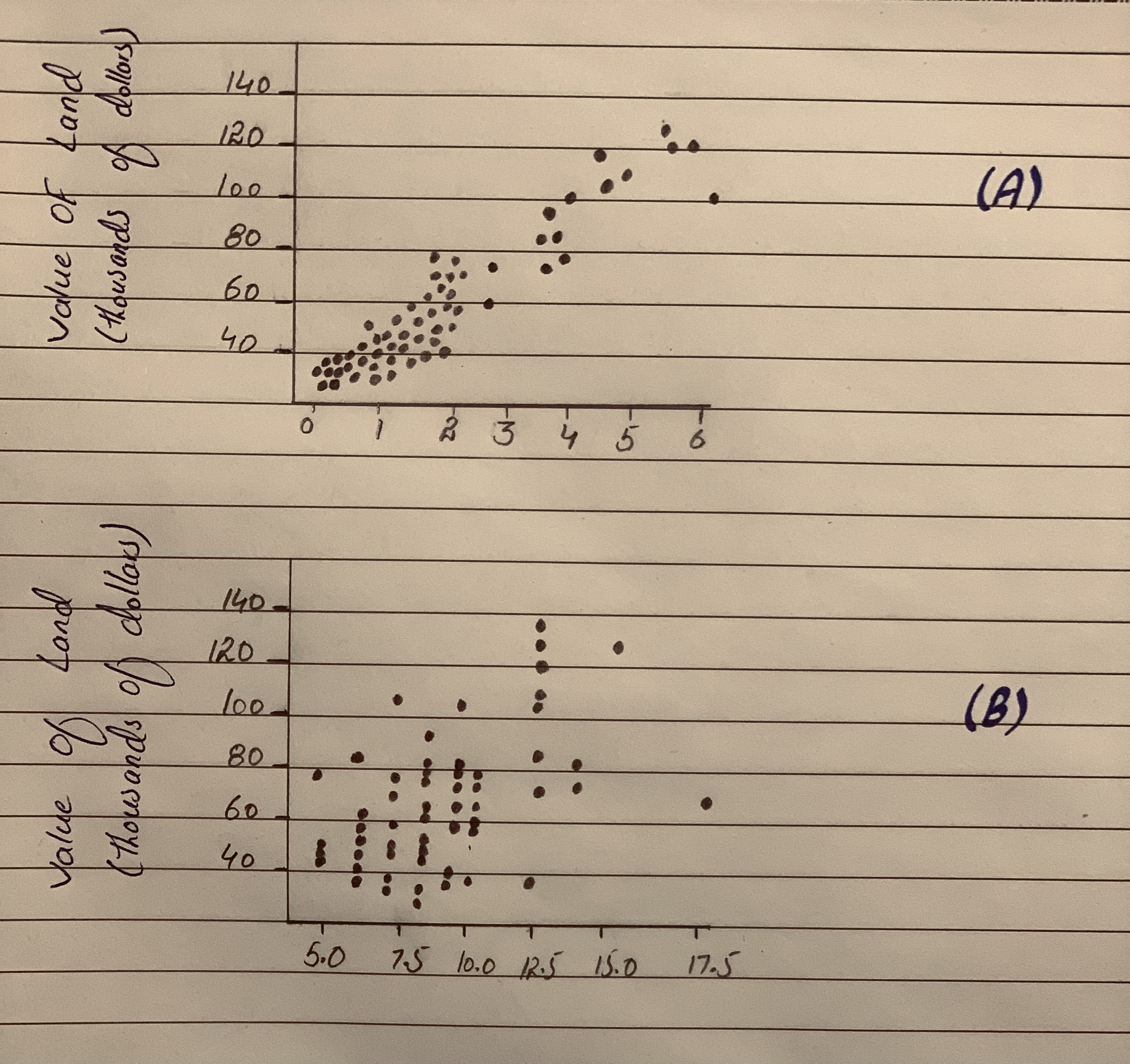Question# Predicting Land Value Both figures concern the assessed value of land (with homes on the land), and both use the same data set. (a). Which do you thin

Scatterplots
ANSWEREDPredicting Land Value Both figures concern the assessed value of land (with homes on the land), and both use the same data set. (a). Which do you think has a stronger relationship with value of the land-the number of acres of land or the number of rooms n the homes? Why? b. Il you were trying to predict the value of a parcel of land in e arca (on which there is a home), would you be able to te a better prediction by knowing the acreage or the num- ber of rooms in the house? Explain. (Source: Minitab File, Student 12. "Assess.")2021-02-09
4.1
The scatterplot (A) is the plot between variables 'acres of land' and 'value of land'. The scatterplot (B) is the plot between variables 'number of rooms' and 'value of land'.
a.
From scatterplot (B) it can be observed that all the data points are not that closer (far away) to each other. Moreover, the data points does not form a linear pattern indicating that the relation between the variables rooms and value of land is not stronger.
The scatterplot (A) has the strongest relationship because all the data points are approximately closer to each other. Also, the data points approximately formed a straight line indicating that there is linear relationship between variables. This shows that, 'acres of land' has the stronger relationship with the 'value of land'.
Thus, 'acres of land' has the stronger relationship with the 'value of land'.
b.
From the two scatterplots it can be observed that, the variable 'acres of land' has the stronger relationship with the 'value of land' when compared to the variable 'rooms'.
If the value of a parcel of land has to be predicted it would be better knowing acreage as there is stronger relationship between variables 'acres of land' and 'value of land'.
Thus, if you were trying to predict the value of a parcel of the land in this area, it would be able to make better prediction by knowing the acreage.